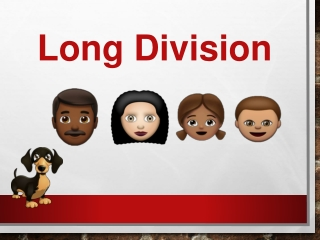Download PresentationLong Division

# Long Division

Download Presentation## Long Division

- - - - - - - - - - - - - - - - - - - - - - - - - - - E N D - - - - - - - - - - - - - - - - - - - - - - - - - - -
##### Presentation Transcript

1. Long Division

2. Overview Long division is as simple as memorizing the people in this family. Each person represents a step in the division process. Dad Mom Sister Brother Step 2: Multiply Step 1: Divide Step 3: Subtract Step 4: Bring Down Rover Step 5: Repeat or Remainder

3. Step 1: Divide 4 Divide the first number in the divisor by the dividend ) 9 4 7 2 • Write that quotient directly above dividenddigit into which you just divided. How many 2’s will go into 9?

4. Step 2: Multiply 4 Multiply the divisor and the first number in the quotient. 2 ) 9 4 7 8 • Write the product directly under the the dividend digit into which you just divided. 2 x 4 = ?

5. Step 3: Subtract 4 Subtract the product from dividend digit into which you just divided. 2 ) 9 4 7 • Draw an equal sign under the 8. 8 1 • Write a subtraction sign next to the 8. • Write the difference directly below the product. 9 - 8 = ?

6. Step 4: Bring Down 4 Bring down the number in the dividend to the right of the first number used. 2 ) 9 4 7 8 • Write an arrow under the 4. • Bring the next number in the dividend down next to the difference. 1 4

7. Step 5: Repeat or Remainder 4 2)9 4 7 This is where you decide whether to repeat the 5 steps of division. 8 • If the divisor can divide into the new number, or if there are numbers in the dividend that have not been brought down, repeat the 5 steps of division. 4 1

8. Step 1: Divide 4 7 2)9 4 7 Divide the new number by the divisor 8 • Write that quotient directly above dividenddigit into which you just divided. 4 1 How many 2’s will go into 14?

9. Step 2: Multiply 7 4 2)9 4 7 Multiply the divisor and the next number in the quotient. 8 • Write the product directly under the the number created when the last digit was brought down. 4 1 4 1 2 x 7 = ?

10. Step 3: Subtract 7 4 Subtract the product from the number created when the last digit was brought down. 2)9 4 7 8 • Draw an equal sign under the 14. 4 1 1 4 • Write a subtraction sign next to the 14. 0 • Write the difference directly below the product. 14 - 14 = ?

11. Step 4: Bring Down 4 7 Bring down the next number in the dividend. 2)9 4 7 8 • Write an arrow under the the next number in the dividend. 4 1 • Bring the next number in the dividend down next to the difference. 1 4 0 7

12. Step 5: Repeat or Remainder 4 7 2)9 4 7 Decide whether to repeat the 5 steps of division. 8 • If the divisor can divide into the new number, or if there are numbers in the dividend that have not been brought down, repeat the 5 steps of division. 4 1 1 4 0 7

13. Step 1: Divide 3 4 7 2)9 4 7 Divide the new number by the divisor 8 • Write that quotient directly above dividenddigit into which you just divided. 4 1 0 7 1 4 How many 2’s will go into 7?

14. Step 2: Multiply 3 4 7 2)9 4 7 Multiply the divisor and the next number in the quotient. 8 • Write the product directly under the the last digit brought down. 4 1 0 7 1 4 6 2 x 3 = ?

15. Step 3: Subtract 3 4 7 Subtract the product from the number created when the dividend digit was brought down. 2)9 4 7 8 • Draw an equal sign under the 6. 4 1 0 7 • Write a subtraction sign next to the 6. 1 4 • Write the difference directly below the product. 6 7 - 6 = ? 1

16. Step 4: Bring Down 3 4 7 2)9 4 7 Bring down the next number in the dividend. 8 • If there are no numbers in the dividend left to bring down, move to step 5. 4 1 1 4 0 7 6 1

17. R1 Step 5: Repeat or Remainder 3 4 7 2)9 4 7 Decide whether to repeat the 5 steps of division. 8 • If the divisor cannot divide into the new number, or if there are no numbers in the dividend that have not been brought down, the difference becomes the remainder. 4 1 0 7 1 4 6 • Write an “R” and the remainder next to the last digit in the quotient. 1

18. R1 3 You did it! 4 7 2)9 4 7 YEAH BABY 8 4 1 0 7 Nice work! 1 4 6 1 YAAAASSS

19. Questions